International
Tables for
Crystallography
Volume B
Reciprocal space
Edited by U. Shmueli

International Tables for Crystallography (2006). Vol. B. ch. 2.1, pp. 194-195   | 1 | 2 |

## Section 2.1.4.3. The central-limit theorem

U. Shmuelia* and A. J. C. Wilsonb

aSchool of Chemistry, Tel Aviv University, Tel Aviv 69 978, Israel, and bSt John's College, Cambridge, England
Correspondence e-mail:  ushmueli@post.tau.ac.il

#### 2.1.4.3. The central-limit theorem

| top | pdf |

A simple form of this important theorem can be stated as follows:

If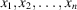are independent and identically distributed random variables, each of them having the same mean m and variance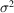, then the sum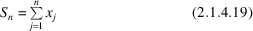tends to be normally distributed – independently of the distribution(s) of the individual random variables – with mean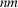and variance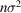, provided n is sufficiently large.

In order to prove this theorem, let us define a standardized random variable corresponding to the sum, i.e., such that its mean is zero and its variance is unity: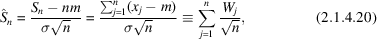where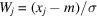is a standardized single random variable. The characteristic function of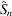is therefore given by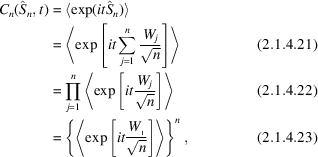where the brackets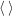denote the operation of averaging with respect to the appropriate probability density function (p.d.f.) [cf. equation (2.1.4.1)]. Equation (2.1.4.22)follows from equation (2.1.4.21)by the assumption of independence, while the assumption of identically distributed variables leads to the identity of the characteristic functions of the individual variables – as seen in equation (2.1.4.23).

On the assumption that moments of all the orders exist – a most plausible assumption in situations usually encountered in structure-factor statistics – we can now expand the characteristic function of a single variable in a power series [cf. equation (2.1.4.10)]: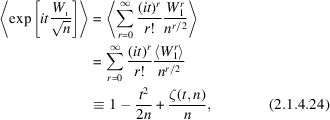since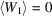,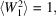and the quantity denoted by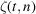in (2.1.4.24)is given by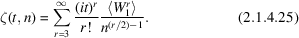The characteristic function ofis therefore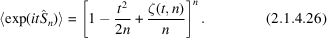Now, as is seen from (2.1.4.25), for every fixed t the quantitytends to zero as n tends to infinity. The cumulant-generating function of the standardized sum then becomes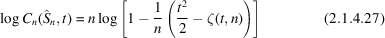and the logarithm on the right-hand side of equation (2.1.4.27)has the form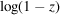with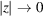as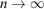. We may therefore use the expansion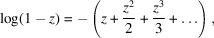which is valid for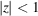. We then obtain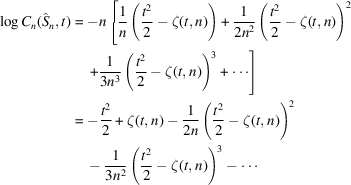and finally, for every fixed t,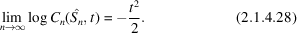Since the logarithm is a continuous function of t, it follows directly that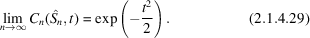The right-hand side of (2.1.4.29)is just the characteristic function of a standardized normal p.d.f., i.e., a normal p.d.f. with zero mean and unit variance [cf. equation (2.1.4.5)]. The asymptotic expression for the p.d.f. of the standardized sum is therefore obtained as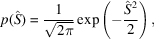which proves the above version of the central-limit theorem.

Surprisingly, this theorem has a very wide applicability and values of n as low as 30 are often large enough for the theorem to be useful. Situations in which the normal p.d.f. must be modified or replaced by an altogether different one are dealt with in Sections 2.1.7and 2.1.8of this chapter.